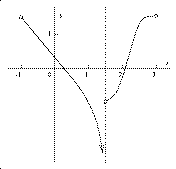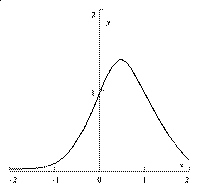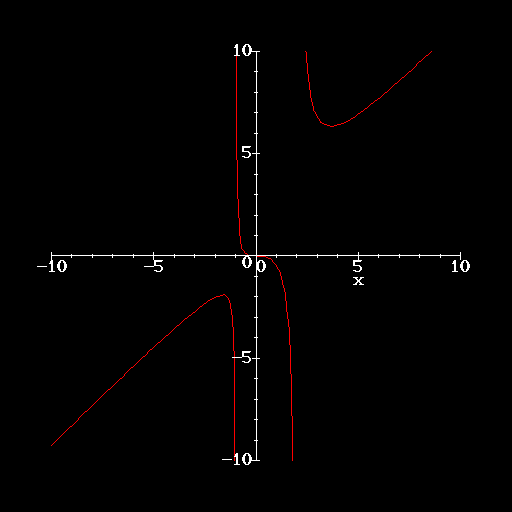Notation exp(Frog) will denote eFrog. sqrt(Toad) will denote the square root of Toad (so sqrt(4) is 2).

Problem 1 You should draw a picture! The candidate rectangles have corners at the points (-x,0) and (x,0) and (-x,4-x2) and (x,4-x2). The lengths of the sides of the rectangle are 2x and 4-x2, so its area A is 8x-2x3. Here x must be between 0 and 2 to get a "real" rectangle. Note that A is 0 when x is 0 or when x is 2, so a positive maximum will occur at a critical number between 0 and 2. A´(x) is 8-6x2. A's critical numbers are 0 and sqrt(4/3). We want the positive critical number since it will supply the maximum because of the reasons stated before. From this you can get the length of the sides (one side has length 2sqrt(4/3) and the other has length 8/3) and area, which is (16/3)sqrt(4/3).Problem 2 The information given in this problem is graphical, so what you can answer is principally qualitative, not quantitative. Here is a graph of h´. We can see that h seems to have two critical numbers. There's one critical number at x=.2 or maybe .3 and there's another at x=2. Also h has a relative maximum at the left-hand critical number and h has a relative minimum at the right-hand critical number. h does not seem to have any inflection points, although to the left of 1.5 the graph is concave down and to the right of 1.5 the graph is concave up, 1.5 itself is not in the domain of h, so h can't have an inflection point with x=1.5 (tricky!).

Problem 3 Suppose the numbers are a and b. Then a+b=P. We are supposed to maximize a3b2: make it largest. We convert this to a problem in calculus of one variable using the constraint a+b=P, or b=P-a. We need to maximize a3(P-a)2 with 0 < a < P. Of course near the endpoints this function gets close to 0. So the maximum will occur where the derivative is 0. The derivative is 3a2(P-a)2 - a32(P-a)1 (the - sign is from the chain rule!). Some simplification is useful and we can factor so the derivative becomes: a2(P-a) (3(P-a)- 2a). The first factor tells us there's vanishing at a and at P (we don't care about these!). The other factor tells us that the derivative is 0 also vanishes at (3/5)P. This gives us a maximum. The endpoint conditions tell us it is a maximum, and this can be checked with the first or second derivative. Select the numbers so that the one to be cubed is three-fifths of the sum, and the one to be squared is two-fifths of the sum.

Problem 4 a) As x->infinity, the largest part is exp(4x). Divide the top and bottom by this, and get on top exp(-2x). The bottom is 3exp(-4x)+1. The limit of the bottom is 1 and the limit of the top is 0 as x->infinity, so the quotient has limit 0. As x->-infinity, the bottom approaches 3 and the top approaches 0. Therefore the limit is 0.
b) Again divide the top and bottom by exp(4x). Now as x->infinity, the top ->1 but the bottom (which is 3exp(-4x)+exp(-2x)) ->0 and is positive. So the quotient ->+infinity. Another way to analyze the behavior is by dividing by exp(2x). As x->-infinity, the limit will be 0 (same reason as in a)).
c) Divide the top and bottom by x2. The top leads off with x, and the bottom leads off with 1 (the non-leading terms are lower order when x->+infinity or -infinity). So the limit as x->+infinity is +infinity and the limit as x->-infinity is -infinity.
d) This one is tricky. The top looks like "x" and a quick glance at the bottom says, well, there's a square root of x2, so it is "x" also (again, neglecting lower order terms). There will be no problem when x->+infinity: the limit is 1. But look again at the bottom, and now algebra snarls at us: remember that the square root of a number squared is the absolute value of the number. Therefore, when x is large NEGATIVE, the bottom is approximately -x, not x! So the quotient is close to -1, and the limit is -1 as x->-infinity. I advise you to graph this function (and also the others) to verify that the claims made here are correct.

Problem 5 You should draw a picture! Here are some variables: the rectangle will have length L and width W and area A and length of a diagonal (they are both equal!) D. Then we know A=LW and D2=L2+W2 (Pythagoras). Yes, we could take the square root, but maybe this is easier to handle.
We also know that at "at certain time" L=3, W=5, L´=+.4 and W´=-.5 (all derivatives are with respect to time). Now let's get to work!
a) The product rule tells us that A´=L´W + LW´ so that at the "certain time" A´ is (.4)5+3(.-5)=.5 and we see that the area is increasing.
b) Implicit differentiation (the chain rule) tells us 2DD´ = 2LL´+2W W´ and at the "certain time" the equation becomes 2DD´=2(3)(.4)+2(5)(-.5)=-2.6: therefore I already know that D is decreasing. The exact amount can only be found if I discover what D is, and for that I use the original equation D2=L2+W2which becomes D2=32+52=34 so D=sqrt(34) and finally D´=-2.6/(2sqrt(34)).Problem 6 a) A graph is presented at the right. Please note the difference in horizontal and vertical scales.
b) f´(x)=exp(x-x2) (1-2x). The only root is x=1/2. f´ is positive to the left of 1/2 and negative to the right of 1/2. Therefore f is increasing in the interval [-2,.5] and decreasing in the interval [.5, 2].
c) f´´(x)=exp(x-x2) (1-2x)2 + exp(s-x2)(-2)=exp(x-x2)(1 -4x+4x2 -2). Since the exponential function is never 0, any roots of f´´(x)=0 are where 1-4x+4x2-2=0 or where 4x2-4x-1=0. The quadratic formula says that these roots are at (+4 +\-sqrt(32))/8=(approximately) 1.21 and -.21 . These turn out to be inflection points of f, because f is concave up between -2 and -.21, concave down between -.21 and 1.21 and finally concave up again between 1.21 and 2.Problem 7 There is one relative maximum, indicated by M on the picture. There is one relative minimum, indicated by m on the picture. There is one inflection point, indicated by I on the picture. The x-axis whose equation is y=0 is a horizontal asymptote and the line x=1 is a vertical asymptote.

Problem 8 Suppose the radius is r and the area is a. Then a=(PI)r2. Taking derivatives with respect to time, we see that a´=2(PI)rr´ (don't forget the chain rule!). At "a certain time" we know r´=.3 and a=25. So 25=(PI)r2 at that time, and we can solve for r, which turns out to be sqrt(25/PI). Therefore since a´=2(PI)rr´ we get a´=2(PI)sqrt(25/PI)(.3)=3sqrt(PI) (wow!).

Problem 9 We will need Q´(x)=3x2+2(x-4). The critical numbers of Q are 4/3 and -2.
a) The easiest way to find the max and min of Q on the interval [-4,4] is to test Q's values at the endpoints and the critical numbers in the interval. So:

Q(-4)=0; Q(4)=64; Q(4/3)=(256)/(27) (approximately 9.48); Q(-2)=28
The max value of Q on this interval is 64 and its min value is 0.
b) Here we need only test Q's values at 0 and 4/3 and 4. Q(0)=16, and the other values are above. So the max value of Q on this interval is 64 and its min value is approximately 9.48.
Check this computation by looking at a graph of Q, please.

Problem 10 a) The only way a quotient can be 0 is if the top is 0. sin(x) is 0 only at integer multiples of PI.
b) Recognition that the function in this part of the problem is the derivative of the function in the first part of the problem is most important. Once you do that, an application of Rolle's Theorem tells you that the derivative must be zero at least once between at two roots of the function. There are at least 6 roots of the function in an interval of length 20, so the derivative must be 0 at least 5 distinct times. There are actually 6 times the derivative is 0, because we're "lucky" - the function is 0 at 0 and the function has 7 roots in [0,20].

Problem 11 If the square bottom has side length S and the height of the box is H (both measured in feet), then the volume is S2H. The constraint is 10=S2H. The "objective function" to be minimized is the cost. The sides have area 4SH and cost \$10 per square foot, so their cost is 40SH. The bottom has area S2 and costs \$20 per square foot, so its cost is 20S2. The total cost is C=40SH+20S2. We use the constraint to reduce this to a problem of only one variable. So we try H=10/(S2) and get C=40S(10/(S2))+20S2=400/S+20S2. Note that when S->0+ or when S->infinity, C gets very large. We have just shown that the minimum cost must occur at a critical point. You could also see this using the first or second derivatives.
What are the critical point(s) of C? C´=-400/S2+40S. We can find out where C´=0 easily, and this happens when S=(10)1/3 which is approximately 2.154 and for this S, the cost is approximately \$278.59 (that is, \$60(102/3).

Problem 12 Repeated from a discussion of an earlier problem: "The information given in this problem is graphical, so what you can answer is principally qualitative, not quantitative."
Q is continuous everywhere except 0, where the left- and right-hand limits emphatically do not coincide. Q seems to be differentiable everywhere except 0. Q seems to have three critical numbers: there are two places where the derivative exists and is 0 (horizontal tangent lines), at about -1.5 and 1, and one place where the derivative does not exist, 0. The y-axis (whose equation is x=0) seems to be a vertical asymptote, and there seem to be two horizontal asymptotes, the x-axis with equation y=0 and the line y=1. Q seems to be increasing in the intervals [-1.5,0] and (0, 1]. Note that Q is NOT increasing in the whole interval [-1.5,1]. This is a bit delicate! Q is decreasing in the intervals (-infinity,-1.5] and [1,infinity). Q seems to be concave up in [-1.5, -.75] and in [1.6, infinity) (your answers may differ - I'm just looking at the graph!) and is concave down in (-infinity, -2] and in [-1,0] and in (0, 1.6). There are three points of inflection: -1.5 and -.75 and 1.6. The limit at -infinity seems to be +1, the limit at 0- seems to be 1.5, the limit at 0+ seems to be -infinity, and the limit at +infinity seems to be 0,

Problem 13 We know 10x+2y+2xy´+4yy´=0 by implicit differentiation from the original equation. So y´=-(10x+2y)/((2x+4y).
a) Horizontal tangent lines occur where y´ is 0. That is where 10+2y=0 or where y=-5x. The original equation is 5x2+2xy + 2y2=7, so we know that 5x2+2x(-5x)+2(-5x)2=7 at points where the tangent lines are horizontal. This is just 45x2=7 whose roots are approximately +/-.394. y is then -5 times each of those, or approximately -/+ 1.97. So the points desired are (.394,-1.97) and (-.394, 1.97).
b) Vertical tangent lines have "no slope". So these occur where 2x+4y=0 or where x=-2y. Then 5x2+2xy+2y2=7 becomes 5(-2y)2+2(-2y)y+2y2=7 which gives 18y2=7, whose roots are approximately +/-.623, so that x must be approximately -/+1.246. The points here are about (-1.246,.623) and (1.246,-.623).
c) If you really believe in the hint, the box "clearly" has corners (1.246,1.97) and (-1.246,1.97 ) and (1.246,-1.97) and (-1.246,-1.97).

Problem 14 This is an intricate problem. Here F(x)=x3/(x2-x-2). Probably the hardest part of this problem (certainly the most irritating part of the problem!) is finding nice forms for F´ and F´´. Here they are:

F´(x)=(x2 (x2 -2x-6) )/ (x2 -x -2)2     F´´(x)= (6x(x2+2x+4))/(x2-x-2)3
Once you've got these, the remainder of the problem involves some algebraic scrambling, but shouldn't be as hard! The domains of F´ and F´´ are the same as the domain of F so relative extrema must occur among points where F´(x)=0 and inflection points must occur among points where F´´(x)=0. If you have not gotten these formulas, please try the problem now with the formulas above before looking at the rest of the answer.

What happens on the function level

Exclusions from the domain of F are found by discovering when the bottom of F's formula is 0. This happens at -1 and 2. The limit of F at +infinity is +infinity and at -infinity is -infinity (look at the highest degree terms of the top and bottom, please). The limits on either side of -1 and 2 of F are gotten by writing F(x) as x3/((x+1)(x-2)). For example, if x is to the left of -1 but close to -1, the formula for F reveals that F(x) is approximately -1/((a small negative number)(-3)) which is a large (in magnitude) negative number, so the limit as x->-1- must be -infinity. The right-hand limit at -1 is +infinity, and the left- and right-hand limits at 2 are -infinity and +infinity respectively. F has no horizontal asymptotes, but it does have two vertical asymptotes: x=-1 and x=2.

What happens on the first derivative level

F´ is equal to 0 where any part of the top is 0. Certainly that occurs when x=0 (the first factor) and the quadratic formula finds the roots of the other factor: x=1+sqrt(7) (approximately 3.6) and x=1-sqrt(7) (approximately -1.6). The derivative does not change sign at 0 but it does at the other two roots. Some care needs to be taken, because the derivative also changes sign as x "crosses" the two numbers not in the domain of F! (Tricky!) F has a relative max at 1-sqrt(7) and a relative min at 1+sqrt(7). 0 is neither a relative max nor a relative min! F is increasing in the intervals (-infinity,1-sqrt(7)] and [1+sqrt(7),+infinity). F is decreasing in the intervals [1-sqrt(7),-1) and (-1,2) and (2,1+sqrt(7)]. Note that F is NOT decreasing in any larger intervals: there is no shorter way to indicate F's behavior!

What happens on the second derivative level

The quadratic formula tells us that the second degree polynomial on the top of F´´ is not zero for any real number. So the only place that the second derivative is 0 is 0. Indeed, the second derivative does change sign there, so that F has an inflection point at 0. But the intervals of concavity are also affected by the places where F doesn't exist -- the holes in the domain of F, because there the bottom of F´´ changes sign. So F is concave up in the intervals (-1,0] and (2,+infinity) and F is concave down in the intervals (-infinity,-1) and [0,2).

And now the picture ...

Sketching a graph of F should now be easy (!). The picture below was produced by a very nice computer program called Maple. Maple is available on most Rutgers systems, including eden). When the management attempted to use a graphing calculator to get a picture of F in the same window (-10 to 10 in both x and y) the result was rather disappointing. You should be able to see all the features of F described above in the picture here.Problem 15 The two parts of V are each positive. The exp(-2x) part goes to +infinity when x->-infinity, and the exp(3x) part goes to +infinity when x->+infinity. Therefore it seems likely the function has a minimum value (let me call it "v"), and that the range of the function is [v,+infinity).
V´(x)=-2(exp(-2x))+3(exp(3x)). This is equal to 0 when -2e-2x +3e3x=0. Multiply this equation by e2x and use properties of exponentials. Then it becomes -2 +3 e5x=0 which is equal to 0 when e5x= 2/3 or when 5x= ln(2/3) or when x=(1/5)ln(2/3). This is the minimum of V because we already know what happens to V(x) when x gets large positive or large negative. What is v=V((1/5)ln(2/3))? Some work with exponentials (a healthy activity!) can give it exactly.
v=(exp(-2(1/5)ln(2/3)))+(exp(3(1/5)ln(2/3)))=(2/3)-2/5+(2/3)3/5= (5/6)32/523/5. Another wow! The last "simplification" is recommended only to those who are very desperate or who enjoy algebraic suffering! I tried graphing V(x) and found a "good" graph difficult to get because the exponentials increase quite rapidly: you try to see what it looks like from -3 to 3. The minimum occurs approximately at (-.081,1.960). This is not obvious!

Problem 16 We know that W(x+h) is approximately W(x)+W´(x)h and here x will be 3 and h will first be +.03 and then -.02. So W(3.03) is approximately 4+2(.03) which is 4.06 and W(2.98) is approximately 4+2(-.02) which is 3.96.
Since W´´(3) is negative, it is likely that the graph of y=W(x) is concave down near 3. The linear approximation used above depends on replacing the "true" value of the function by its value on the tangent line. When the graph is concave down, the tangent line is above the graph. It is therefore likely that the approximate values given above are more than the true values of W.